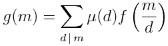Over a million developers have joined DZone.{{announcement.body}}
{{announcement.title}}

# Number Theory Determinant and SymPy

DZone 's Guide to

# Number Theory Determinant and SymPy

· Java Zone ·
Free Resource

Comment (0)

Save
{{ articles.views | formatCount}} Views

Let σ(n) be the sum of the positive divisors of n and let gcd(a, b) be the greatest common divisor of a and b.

Form an n by n matrix M whose (i, j) entry is σ(gcd(i, j)). Then the determinant of M is n!.

The following code shows that the theorem is true for a few values of n and shows how to do some common number theory calculations in SymPy.

from sympy import gcd, divisors, Matrix, factorial

def f(i, j):
return sum( divisors( gcd(i, j) ) )

def test(n):
r = range(1, n+1)
M = Matrix( [ [f(i, j) for j in r] for i in r] )
return M.det() - factorial(n)

for n in range(1, 11):
print test(n)

As expected, the test function returns zeros.

If we replace the function σ above by τ where τ(n) is the number of positive divisors of n, the corresponding determinant is 1. To test this, replace sum by len in the definition of f and replace factorial(n) by 1.

In case you’re curious, both results are special cases of the following more general theorem. I don’t know whose theorem it is. I found it here.

For any arithmetic function f(m), let g(m) be defined for all positive integers m byLet M be the square matrix of order n with ij element f(gcd(i, j)). ThenHere μ is the Möbius function. The two special cases above correspond to g(m) = m and g(m) = 1.

Topics:

Comment (0)

Save
{{ articles.views | formatCount}} Views

Published at DZone with permission of

Opinions expressed by DZone contributors are their own.